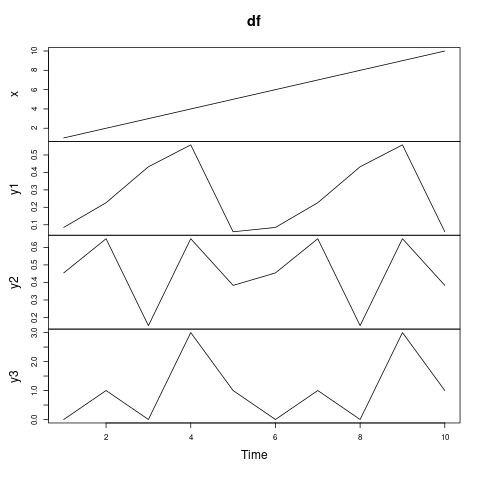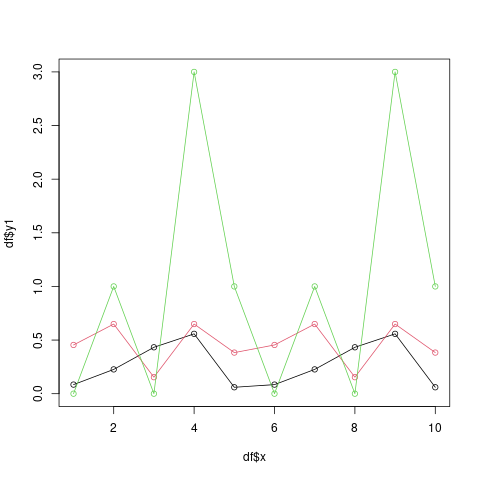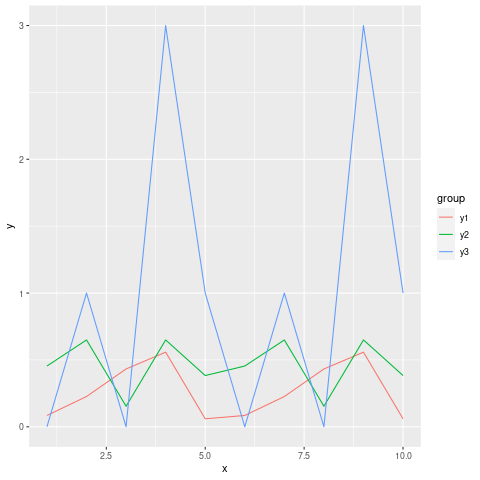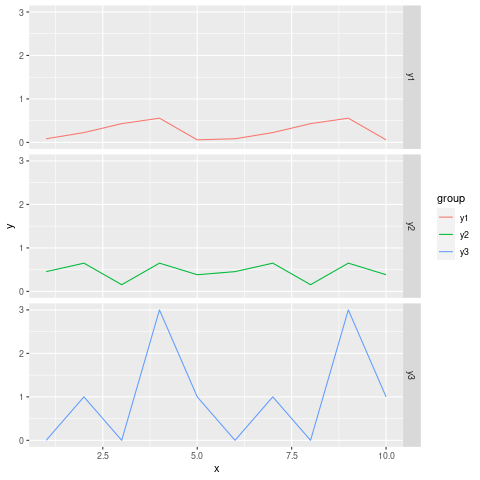Open in App
Not now

# How to plot all the columns of a dataframe in R ?

• Last Updated : 10 Sep, 2021

In this article, we will learn how to plot all columns of the DataFrame in R programming language.

Dataset in use:

``` x         y1        y2 y3
1   1 0.08475635 0.4543649  0
2   2 0.22646034 0.6492529  1
3   3 0.43255650 0.1537271  0
4   4 0.55806524 0.6492887  3
5   5 0.05975527 0.3832137  1
6   6 0.08475635 0.4543649  0
7   7 0.22646034 0.6492529  1
8   8 0.43255650 0.1537271  0
9   9 0.55806524 0.6492887  3
10 10 0.05975527 0.3832137  1```

### Method 1: using plot.ts() function

We just have to pass our dataframe in the plot.ts() function, and it will plot all the dataframe columns in a time series plot. In the Y-axis we can see the names of the columns of our dataframe.

Syntax: plot.ts(df)

Parameters:

df: DataFrame object

Example:

## R

 `set.seed``(200)                                            ` `df <- ``data.frame``(x = 1:10,``                   ``y1 = ``rnorm``(5),``                   ``y2 = ``runif``(5),``                   ``y3 = ``rpois``(5, 1))``plot.ts``(df)`

Output:### Method 2: using zoo() function

The zoo() function is present in the zoo package, so it has to be imported by placing require(zoo) function on the first line of the code. This function converts the dataframe in such a format(“zoo” series) that it can be plotted easily in a form of a time series plot.

Since y is NULL in our case, a time series plot of x is produced. But if both x and y were a uni-variate “zoo” series then a scatter plot of y vs x would’ve been produced.

Syntax: plot(zoo(df))

Parameters:

df: DataFrame object

Example:

## R

 `require``(zoo)``set.seed``(200)                                            ` `df <- ``data.frame``(x = 1:10,``                   ``y1 = ``rnorm``(5),``                   ``y2 = ``runif``(5),``                   ``y3 = ``rpois``(5, 1))` `df <- ``zoo``(df)``plot``(df)`

Output:### Method 3: using plot() function

In this approach, we will plot a line chart showing multiple columns of a data frame using the basic plot() function. The plot() function is defined as a generic function for plotting in R Language. It can be used to create basic plots of a different type.

Syntax: plot(X, Y, type = “l”, col = 1, ylim = c(0, 3))

Parameters:

X: X-axis. ->

Y: Y-axis ->

type: specifies the type of plot

col: specifies colour

ylim: specifies limit for y-axis

In the code below first dataframe column is X-axis, and the rest columns are y-axis, and they are plotted against the first column in form of a line chart. The value of col should be different for different lines such that different line has a different color, and finally we have specified ylim which limits the value of y-axis.

We can also plot different types of plots by specifying different values for type parameters. For example, type=”h”, would have plotted a histogram.

Example:

## R

 `require``(zoo)``set.seed``(200)                                            ``df <- ``data.frame``(x = 1:10,``                   ``y1 = ``rnorm``(5),``                   ``y2 = ``runif``(5),``                   ``y3 = ``rpois``(5, 1))` `plot``(df\$x, df\$y1, type = ``"o"``, col = 1, ylim = ``c``(0, 3))``lines``(df\$x, df\$y2, type = ``"o"``, col = 2)``lines``(df\$x, df\$y3, type = ``"o"``, col = 3)`

Output:### Method 4: Using ggplo2() package

For this the data has to be reshaped to be in the form where it can be plotted.

Example:

## R

 `library``(ggplot2)` `set.seed``(200)                                            ``df <- ``data.frame``(x = 1:10,``                   ``y1 = ``rnorm``(5),``                   ``y2 = ``runif``(5),``                   ``y3 = ``rpois``(5, 1))` `# Reshape data frame``df_reshaped <- ``data.frame``(x = df\$x,                           ``                       ``y = ``c``(df\$y1, df\$y2, df\$y3),``                       ``group = ``c``(``rep``(``"y1"``, ``nrow``(df)),``                                 ``rep``(``"y2"``, ``nrow``(df)),``                                 ``rep``(``"y3"``, ``nrow``(df))))``head``(df_reshaped,10)`

Output:

```  x          y group
1   1 0.08475635    y1
2   2 0.22646034    y1
3   3 0.43255650    y1
4   4 0.55806524    y1
5   5 0.05975527    y1
6   6 0.08475635    y1
7   7 0.22646034    y1
8   8 0.43255650    y1
9   9 0.55806524    y1
10 10 0.05975527    y1```

Plotting multiple variables in the same panel

In this method, we plot a line graph for each column of the dataframe in the same panel. This can be useful when comparing columns that store same type of data but differ in some nature. After reshaping the data, let us see how line plots of the various column will appear.

Syntax:

ggplot(df_reshaped, aes(x, y, col = group)) +  geom_line()

Example:

## R

 `library``(ggplot2)` `set.seed``(200)                                            ``df <- ``data.frame``(x = 1:10,``                   ``y1 = ``rnorm``(5),``                   ``y2 = ``runif``(5),``                   ``y3 = ``rpois``(5, 1))` `# Reshape data frame``df_reshaped <- ``data.frame``(x = df\$x,                           ``                       ``y = ``c``(df\$y1, df\$y2, df\$y3),``                       ``group = ``c``(``rep``(``"y1"``, ``nrow``(df)),``                                 ``rep``(``"y2"``, ``nrow``(df)),``                                 ``rep``(``"y3"``, ``nrow``(df))))` `ggplot``(df_reshaped, ``aes``(x, y, col = group)) +  ``geom_line``()`

Output:Plotting multiple variables in the different panels

In this method, we plot a line graph for each column of the dataframe in the different panel of a same plot. We can achieve this task by adding facet_grid() function. facet_grid() function produces a layout panel defined by rows and columns.

Syntax:

ggplot(df_reshaped, aes(x, y, col = group)) +  geom_line()+ facet_grid(group ~ .)

Example:

## R

 `library``(ggplot2)` `set.seed``(200)                                            ``df <- ``data.frame``(x = 1:10,``                   ``y1 = ``rnorm``(5),``                   ``y2 = ``runif``(5),``                   ``y3 = ``rpois``(5, 1))` `# Reshape data frame``df_reshaped <- ``data.frame``(x = df\$x,                           ``                       ``y = ``c``(df\$y1, df\$y2, df\$y3),``                       ``group = ``c``(``rep``(``"y1"``, ``nrow``(df)),``                                 ``rep``(``"y2"``, ``nrow``(df)),``                                 ``rep``(``"y3"``, ``nrow``(df))))` `ggplot``(df_reshaped, ``aes``(x, y, col = group)) +  ``geom_line``()+ ``facet_grid``(group ~ .)`

Output:My Personal Notes arrow_drop_up